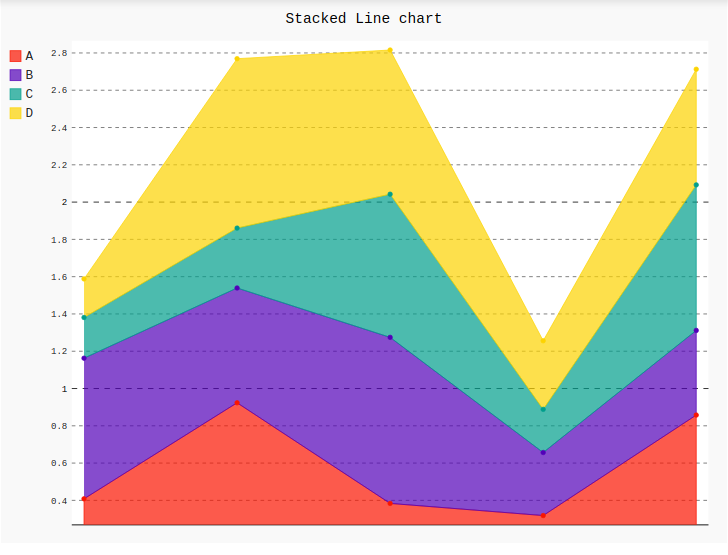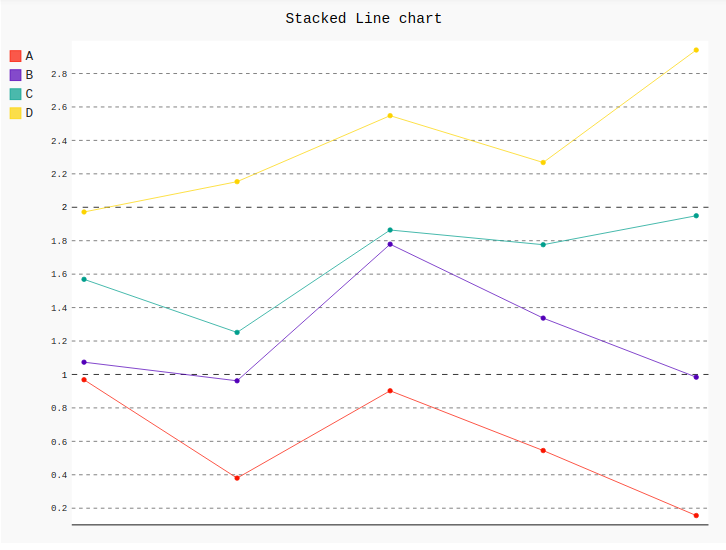# Stacked Line chart in Pygal

Pygal is a Python module that is mainly used to build SVG (Scalar Vector Graphics) graphs and charts. SVG is a vector-based graphics in the XML format that can be edited in any editor. Pygal can create graphs with minimal lines of code that can be easy to understand and write.

## Stacked line chart

A stacked line chart is a line chart in which lines never overlap because they amplify to each other points. Values are represented on the y-axis and categories are displayed on the x-axis. But the stacked line chart cannot present positive and negative value at the same time. Rendering helps to represent the graph more effectively.

Syntax:

`line_chart = pygal.StackedLine(fill=True)`

Example 1:

 `# importing pygal ` `import` `pygal ` `import` `numpy ` ` `  ` `  `# creating line chart object ` `line_chart ``=` `pygal.StackedLine(fill ``=``"True"``) ` ` `  `# naming the title ` `line_chart.title ``=` `'Stacked Line chart'` ` `  ` `  `# adding lines ` `line_chart.add(``'A'``, numpy.random.rand(``5``)) ` `line_chart.add(``'B'``, numpy.random.rand(``5``)) ` `line_chart.add(``'C'``, numpy.random.rand(``5``)) ` `line_chart.add(``'D'``, numpy.random.rand(``5``)) ` ` `  `line_chart `

Output:Example 2:

 `# importing pygal ` `import` `pygal ` `import` `numpy ` ` `  ` `  `# creating line chart object ` `line_chart ``=` `pygal.StackedLine() ` ` `  `# naming the title ` `line_chart.title ``=` `'Stacked Line chart'` ` `  ` `  `# adding lines ` `line_chart.add(``'A'``, numpy.random.rand(``5``)) ` `line_chart.add(``'B'``, numpy.random.rand(``5``)) ` `line_chart.add(``'C'``, numpy.random.rand(``5``)) ` `line_chart.add(``'D'``, numpy.random.rand(``5``)) ` ` `  `line_chart `

Output:My Personal Notes arrow_drop_upIf you like GeeksforGeeks and would like to contribute, you can also write an article using contribute.geeksforgeeks.org or mail your article to contribute@geeksforgeeks.org. See your article appearing on the GeeksforGeeks main page and help other Geeks.

Please Improve this article if you find anything incorrect by clicking on the "Improve Article" button below.

Article Tags :

Be the First to upvote.

Please write to us at contribute@geeksforgeeks.org to report any issue with the above content.